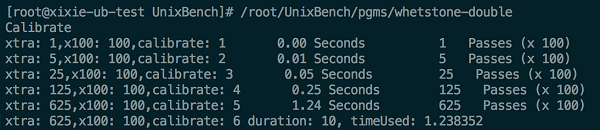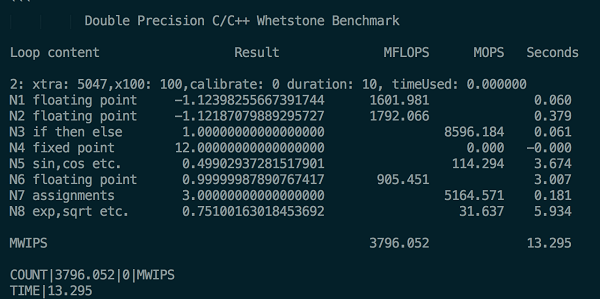×
Community Blog UnixBench: A Detailed Implementation

UnixBench: A Detailed Implementation

This article describes the tests performed by UnixBench from the code level.

By Chao Qian (Xixie)

Many users use UnixBench to compare and to test the performance of VMs provided by different vendors. This article describes the tests performed by UnixBench from the code level.

Before we go into the details of UnixBench implementation, let's check the article UnixBench Score: An Introduction for similar results. From the results, we can see that UnixBench tests consist of two parts: single-process tests and multi-process tests. The number of processes in multi-process tests depends by default on the number of CPUs. The only difference between single-process tests and multi-process tests is the number of processes. Therefore, the following description focuses on single-process tests.

Dhrystone 2 Using Register Variables

Dhrystone is a synthetic computing benchmark program that primarily tests the integer performance of a CPU. The corresponding floating-point number test is Double-Precision Whetstone.

According to the online articles, these unintelligible operations improve the performance through compilation optimization and cannot truly reflect the CPU performance. This article goes into detail about this: Benchmarking in context: Dhrystone.

Let's skip over the operations and look at the output: The test calculates the number of operations done within 10 seconds and obtains the index based on the score according to the first article.

Double-Precision Whetstone

In addition to the tests showing the CPU performance in integer operations, whets.c demonstrates the floating-point number computation performance, which features much higher code quality and intelligibility.

1. It requires an appropriate parameter. How is the operation obtained? In this operation set, when the input parameter (xtra) increases, the computation time consumed gradually increases or decreases. When the time consumed exceeds two seconds, the parameter stops increasing.2. Then, what is the approximate parameter when the computation period is 10 seconds? 625*10/1.238352=5047
3. The results are calculated based on the input parameter. This calculation involves eight steps in total. The floating-point number score is used. However, it also calculates the time consumed by other operations. Though we only care about floating-point number operations, steps N3, N4, N5, N7, and N8 are added. For details about the subsequent computations, see the algorithm rules described in the first article.Execl Throughput

In addition to the two complex operations described above, other UnixBench operations are relatively simple. In Execl, it is actually a recursive call of the execl function. The execution file compiled by execl.c is a binary file of execl. When the execl function is executed, these parameters are recorded: start time, number of executions, and time consumed (generally 10 seconds). The concept is rather clever: The total number of executions is output when the time consumed exceeds 10 seconds, based on which the score is calculated according to the scoring rule.

File Copy

This test mainly checks the write and read functions and takes 30 seconds. Its implementation is simple. First, the code writes a file for two seconds (cyclically) and reads the file for data for two seconds. The data obtained is then written to another file cyclically. In this way, the code obtains the number of read and write operations in 30 seconds. The parameters are used to test the performance with different block sizes. To test disks, FIO is recommended.

Pipe Throughput

This test opens a pipeline, writes 512 bytes to the pipeline, and then reads the data from the pipeline. The test calculates the number of read and write operations in 10 seconds.

Pipe-based Context Switching

This test opens two pipelines and enables two processes. One process writes data to pipeline 1 and reads data from pipeline 2. The other process writes data to pipeline 2 and reads data from pipeline 2. Each time a process completes one read and write cycle, the result increases by 1. Interestingly, the test result is much better if the two processes are performed on the same CPU rather than on different CPUs. The following article in this series will provide a detailed analysis of this issue.

Process Creation

This test repeatedly calls the fork function to create a process and then immediately exits the process. Each time the operation cycle is completed, the result increases by 1.

Shell Scripts

This test uses the fork function to create a process and execute a script repeatedly. Each time the script is executed successfully, the result increases by 1. Shell Scripts (1 concurrent) indicates that the pgms/multi.sh parameter input to the script is 1. Shell Scripts (8 concurrent) indicates that the pgms/multi.sh parameter input to the script is 8 and eight subtasks are executed concurrently.

This test calculates the overhead for entering and exiting the operating system. Each time entry and exit is performed, the result increases by 1. The test calculates the number of executions within 10 seconds. The execution is based on the fork child process. Each time the waitpid function is exited, the result increases by 1.

These are the default implementations of UnixBench, which are very simple but interesting!

0 0 0
Share on

Related Products

•ECS(Elastic Compute Service)

An online computing service that offers elastic and secure virtual cloud servers to cater all your cloud hosting needs.

•Starter Package

Easy-to-use high-performance virtual machines with data transfer plan starting from \$2.50 a month

•GPU(Elastic GPU Service)

Powerful parallel computing capabilities based on GPU technology.

•Batch Compute

Resource management and task scheduling for large-scale batch processing